# Decision tree

23 Feb 2020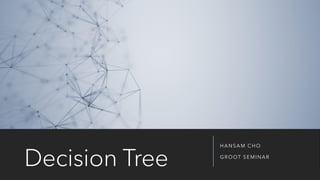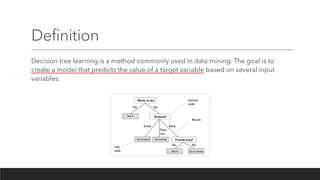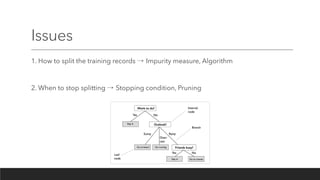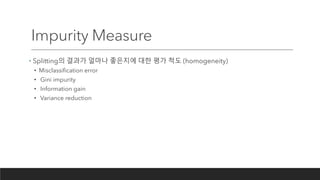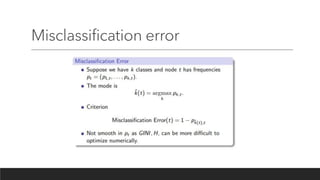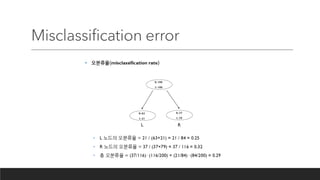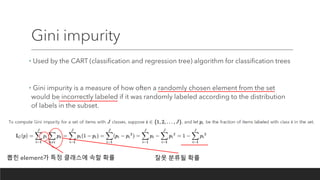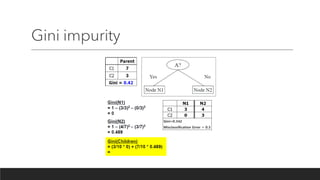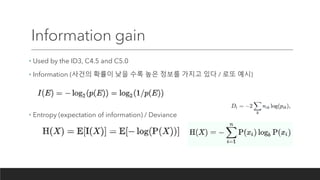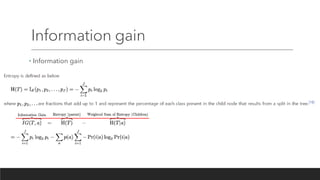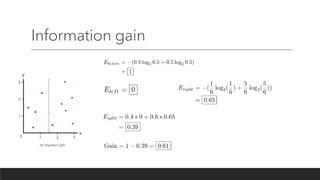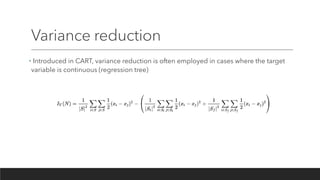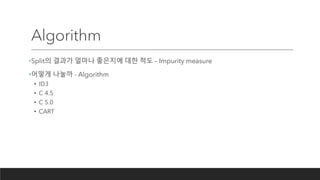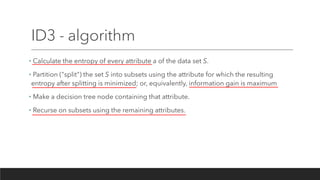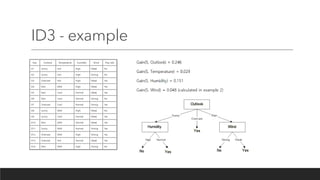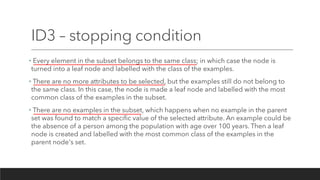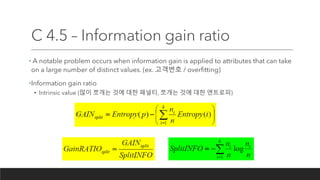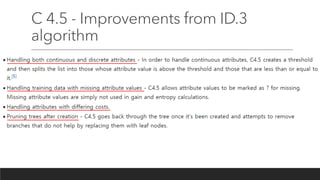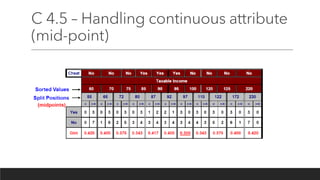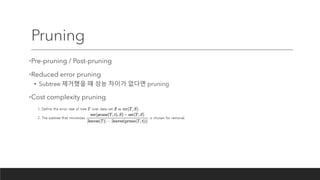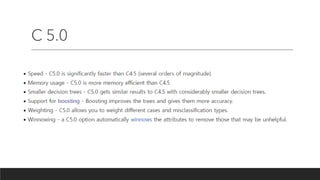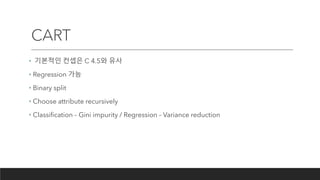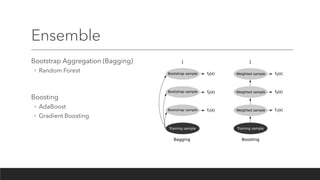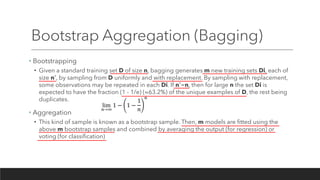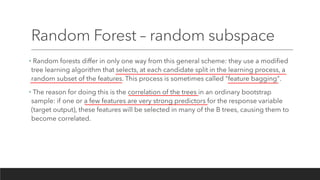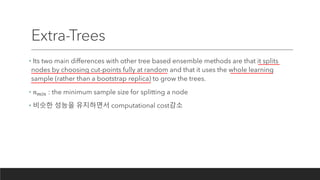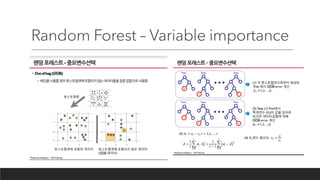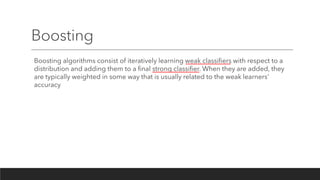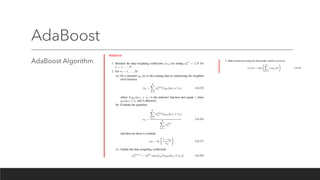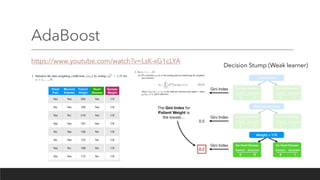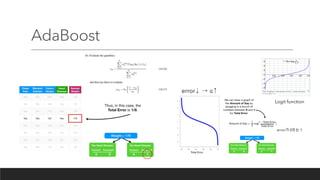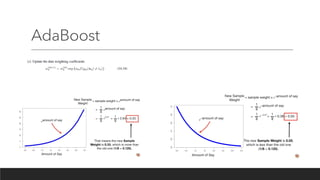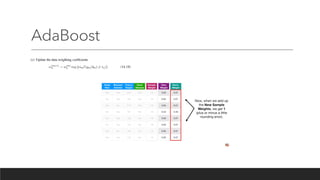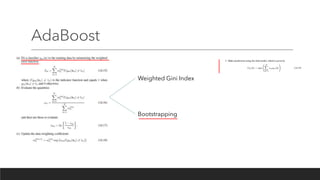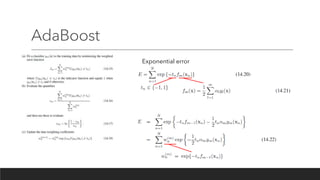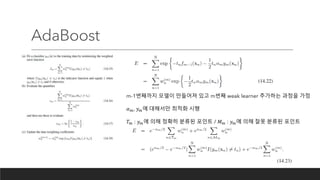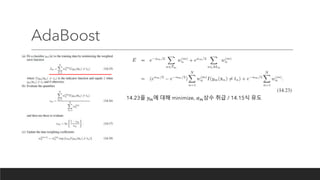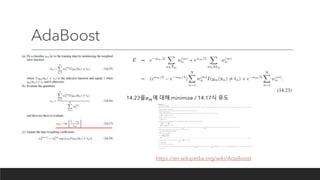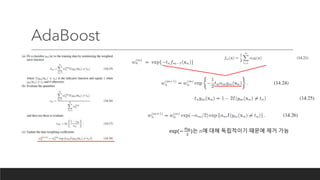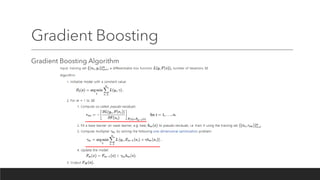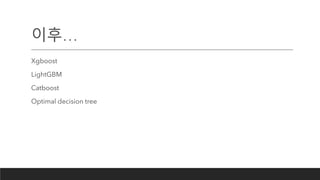1 sur 41

### Decision tree

• 1. Decision Tree HANSAM CHO GROOT SEMINAR
• 2. Definition Decision tree learning is a method commonly used in data mining. The goal is to create a model that predicts the value of a target variable based on several input variables.
• 3. Issues 1. How to split the training records → Impurity measure, Algorithm 2. When to stop splitting → Stopping condition, Pruning
• 4. Impurity Measure • Splitting의 결과가 얼마나 좋은지에 대한 평가 척도 (homogeneity) • Misclassification error • Gini impurity • Information gain • Variance reduction
• 5. Misclassification error
• 6. Misclassification error
• 7. Gini impurity • Used by the CART (classification and regression tree) algorithm for classification trees • Gini impurity is a measure of how often a randomly chosen element from the set would be incorrectly labeled if it was randomly labeled according to the distribution of labels in the subset. 뽑힌 element가 특정 클래스에 속할 확률 잘못 분류될 확률
• 8. Gini impurity
• 9. Information gain • Used by the ID3, C4.5 and C5.0 • Information (사건의 확률이 낮을 수록 높은 정보를 가지고 있다 / 로또 예시) • Entropy (expectation of information) / Deviance
• 10. Information gain • Information gain
• 11. Information gain
• 12. Variance reduction • Introduced in CART, variance reduction is often employed in cases where the target variable is continuous (regression tree)
• 13. Algorithm •Split의 결과가 얼마나 좋은지에 대한 척도 – Impurity measure •어떻게 나눌까 - Algorithm • ID3 • C 4.5 • C 5.0 • CART
• 14. ID3 - algorithm • Calculate the entropy of every attribute a of the data set S. • Partition ("split") the set S into subsets using the attribute for which the resulting entropy after splitting is minimized; or, equivalently, information gain is maximum • Make a decision tree node containing that attribute. • Recurse on subsets using the remaining attributes.
• 15. ID3 - example
• 16. ID3 – stopping condition • Every element in the subset belongs to the same class; in which case the node is turned into a leaf node and labelled with the class of the examples. • There are no more attributes to be selected, but the examples still do not belong to the same class. In this case, the node is made a leaf node and labelled with the most common class of the examples in the subset. • There are no examples in the subset, which happens when no example in the parent set was found to match a specific value of the selected attribute. An example could be the absence of a person among the population with age over 100 years. Then a leaf node is created and labelled with the most common class of the examples in the parent node's set.
• 17. C 4.5 – Information gain ratio • A notable problem occurs when information gain is applied to attributes that can take on a large number of distinct values. (ex. 고객번호 / overfitting) •Information gain ratio • Intrinsic value (많이 쪼개는 것에 대한 패널티, 쪼개는 것에 대한 엔트로피)
• 18. C 4.5 - Improvements from ID.3 algorithm
• 19. C 4.5 – Handling continuous attribute (mid-point)
• 20. Pruning •Pre-pruning / Post-pruning •Reduced error pruning • Subtree 제거했을 때 성능 차이가 없다면 pruning •Cost complexity pruning
• 21. C 5.0
• 22. CART • 기본적인 컨셉은 C 4.5와 유사 • Regression 가능 • Binary split • Choose attribute recursively • Classification – Gini impurity / Regression – Variance reduction
• 23. Ensemble Bootstrap Aggregation (Bagging) ◦ Random Forest Boosting ◦ AdaBoost ◦ Gradient Boosting
• 24. Bootstrap Aggregation (Bagging) • Bootstrapping • Given a standard training set D of size n, bagging generates m new training sets Di, each of size n′, by sampling from D uniformly and with replacement. By sampling with replacement, some observations may be repeated in each Di. If n′=n, then for large n the set Di is expected to have the fraction (1 - 1/e) (≈63.2%) of the unique examples of D, the rest being duplicates. • Aggregation • This kind of sample is known as a bootstrap sample. Then, m models are fitted using the above m bootstrap samples and combined by averaging the output (for regression) or voting (for classification) lim 𝑛→∞ 1 − 1 − 1 𝑛 𝑛
• 25. Random Forest – random subspace • Random forests differ in only one way from this general scheme: they use a modified tree learning algorithm that selects, at each candidate split in the learning process, a random subset of the features. This process is sometimes called "feature bagging". • The reason for doing this is the correlation of the trees in an ordinary bootstrap sample: if one or a few features are very strong predictors for the response variable (target output), these features will be selected in many of the B trees, causing them to become correlated.
• 26. Extra-Trees • Its two main differences with other tree based ensemble methods are that it splits nodes by choosing cut-points fully at random and that it uses the whole learning sample (rather than a bootstrap replica) to grow the trees. • 𝑛 𝑚𝑖𝑛 : the minimum sample size for splitting a node • 비슷한 성능을 유지하면서 computational cost감소
• 27. Random Forest – Variable importance
• 28. Boosting Boosting algorithms consist of iteratively learning weak classifiers with respect to a distribution and adding them to a final strong classifier. When they are added, they are typically weighted in some way that is usually related to the weak learners' accuracy